# Mechquest Trainer Hack / Cheat - SpookMQ

This Mechquest Trainer cheat features
• Star Captain Hack
• DragonFable Weaponry
• Finish Quest
• End Battle
• Stats
• Plus more hacks
How to use this Mechquest hack
2. Run the program called spookMQ 7.0.exe
3. In the swf field, type in 3_9_11 (Note: The swf number can change so if the hack is not working then post a comment and I'll update the number)Anonymous said... on

ty :DAnonymous said... on

doesn't work!!!! swfAnonymous said... on

this is so stupid,3_7_4 doesnt work at all,i got .net 3.5 too,what the heck do i need to do?Anonymous said... on

=)) =)) =)) =)) =)) =)) =)) =)) =)) =)) =)) =)) =)) =)) =)) =)) =)) =)) =)) =)) =)) =)) =)) =)) =)) =)) =)) =)) =)) =)) =)) =)) =)) =)) =)) =)) =)) =)) =)) =)) =)) =)) =)) =)) =)) =)) =)) =)) =)) =)) =)) =)) =)) =)) =)) =)) =)) =)) =)) =)) =)) =)) =)) =)) =)) =)) =)) =)) =)) =)) =)) =)) =)) =)) =)) =)) =)) =)) =)) =)) =)) =)) =)) =)) =)) =)) =)) =)) =)) =)) =)) =)) =)) =)) =)) =)) =)) =)) =)) =)) =)) =)) =)) =)) =)) =)) =)) =))Anonymous said... on

Plz give new numberAnonymous said... on

didnt workAnonymous said... onAnonymous said... on

To find the number go on actual mechquest website click play and before you login look just below the mechquest sign.

Should say: Omega Ver. 3.8.0 or whatever it is.Anonymous said... on

awAnonymous said... on

ive downloaded so many trainers and none work i hope tis does!!!!!:(( :(( :-/Anonymous said... on

new number it sez

ERROR!!!!!:-LAnonymous said... onAnonymous said... on

I'm Using Visual basic 6 How Do I Find Shop Loader Code

Call ShockWaveFlash1.SetVariable What?

Can Anyone Anwer

Unknown said... on

work for me...thank :DAnonymous said... on

current code is 3_9_22 and there is a limit for being found but i dunno what it is. there is also a limit to how much you can get as it saves it as a daily file and they only have so much room in the file.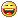:))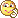;))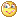;;)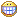:D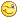;)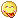:p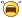:((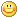:)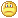:(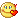:X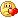=((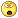:-o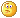:-/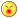:-*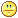:|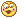8-}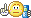:)]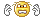~x(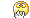:-tb-(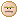:-L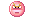x(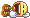=))